Next: Implementation Up: Lagrange-MacLaurin theorem Previous: Lagrange-MacLaurin theorem   Contents

Mathematical background

Let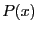be an univariate polynomial of degree: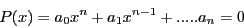Assume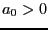and let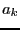(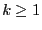) be the first negative coefficients of(ifhas no negative coefficients then there is no positive real root).

The upper bound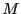of the value of the positive real root is: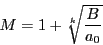where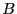is the greatest absolute value of the negative coefficients of,,.

If we define: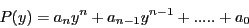Then the upper bound of the positive real roots of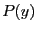is the lower bound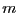of the positive real root of. Consequently if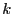andare computed for the polynomialthen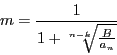Jean-Pierre Merlet 2012-12-20